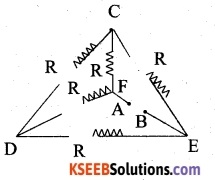# 2nd PUC Physics Question Bank Chapter 3 Current Electricity

You can Download Chapter 3 Current Electricity Questions and Answers, Notes, 2nd PUC Physics Question Bank with Answers, Karnataka State Board Solutions help you to revise complete Syllabus and score more marks in your examinations.

## Karnataka 2nd PUC Physics Question Bank Chapter 3 Current Electricity

### 2nd PUC Physics Current Electricity NCERT Text Book Questions and Answers

Question 1.
The storage battery of a car has an emf of 12V. If the internal resistance of the battery is 0.4 q, what is the maximum current that can be drawn from the Battery?
E = 12V
r = 0.4 Ω
Maximum current is drawn when external resistance of the circuit is zero, i.e. R = 0
∴ $${ \quad I }_{ max }=\frac { E }{ r } =\frac { 12 }{ 0.4 } =30A$$

Question 2.
A battery of emf 10 V and internal resistance 30 is connected to a resistor. If the current in the circuit is 0.5 A, what is the resistance of the resistor? What is the terminal voltage of the battery when the circuit is closed?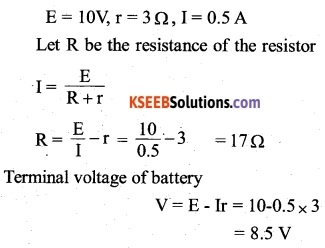Question 3.
(a) Three resistors 1Ω, 2Ω, and 3Ω. are combined in series. What is the total resistance of the combination?
(b) If the combination is connected to a battery of emf 12 V and negligible internal resistance, obtain the potential drop across each resistor.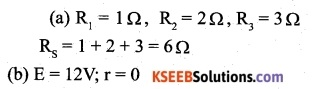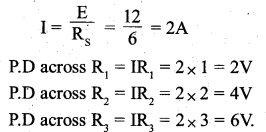Question 4.
(a) Three resistors 2Ω, 4Ω and 5Ω are combined in parallel. What is the total resistance of the combination?
(b) If the combination is connected to a battery of emf 20 V and negligible internal resistance, determine the current through each resistor, and the total current drawn from the battery.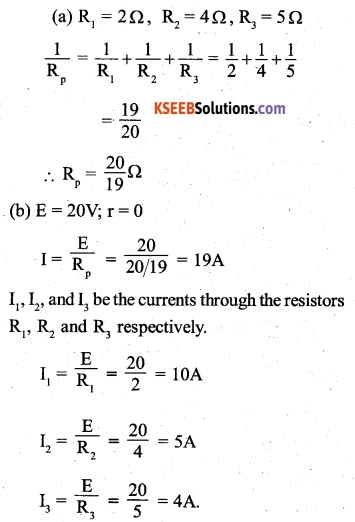Question 5
At room temperature (27.0°C) the resistance of a heating element is 100 Ω What is the temperature of the element if the resistance is found to be 117Ω, given that the temperature coefficient of the material of the resistor is 1.70 x 10-4 °C-1.
R1 =100Ω, θ1 = 27°C, R2 = 117Ω,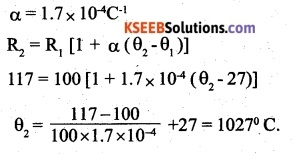Question 6.
A negligibly small current is passed  through a wire of length 15 m and uniform cross-section 6.0 × 10-7 m2, and its resistance is measured to be 5.0 Ω. What is the resistivity of the material at the temperature of the experiment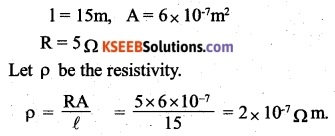Question 7.
A silver wire has a resistance of 2.1Ω, at- 27.5 °C, and a resistance of 2.7Ω, at 100 °C. Determine the temperature coefficient of resistivity of silver.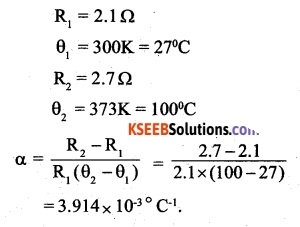Question 8.
A heating element using nichrome connected to a 230 V supply draws an initial current of 3.2 A which settles after a few seconds to a steady value of 2.8 A. What is the steady temperature of the heating element if the room temperature is 27.0 °C? Temperature coefficient of resistance of nichrome averaged over the temperature range involved is 1.70 ×10-4 °C-1
V = 230 volt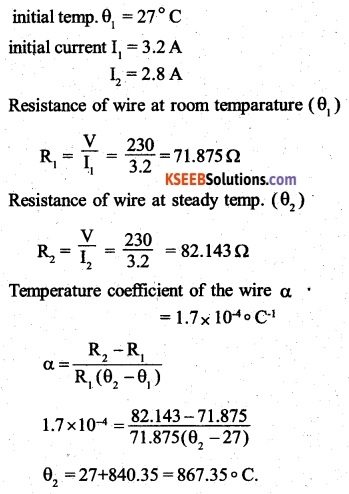Question 9.
Determine the current in each branch of the network shown in F1&. 3.30: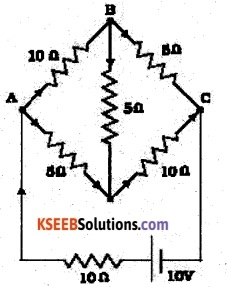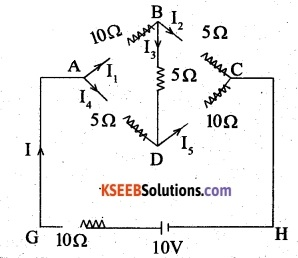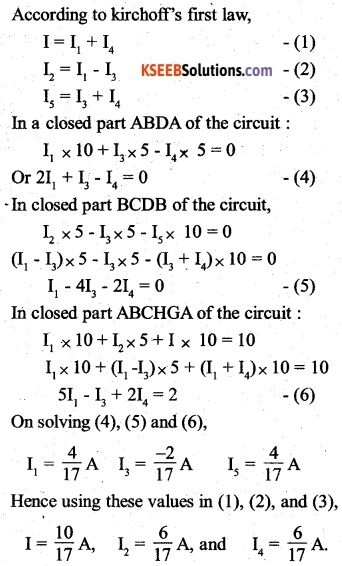Question 10.
(a) In a metre bridge [Fig. 3.27], the balance point is found to be at 39.5 cm from the end A, when the resistor Y is of 12.5 ft Determine the resistance of X. Why are the connections between resistors in a Wheatstone or meter bridge made of thick copper strips?
(b) Determine the balance point of the bridge above if X and Y are interchanged.
(c) What happens if the galvanometer and cell are interchanged at the balance point of the bridge? Would the galvanometer show any current?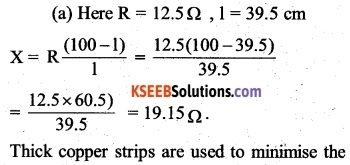resistance of the stripes, as the resistance of connecting copper strips, has not been accounted to be used in this formula for unknown resistance.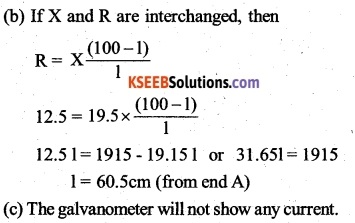Question 11.
A storage battery of emf 8.0 V and internal resistance 0.5 is being charged-by a 120 V dc supply using a series resistor of 15.5 Q. What is the terminal voltage of the battery during charging? What is the purpose of having a series resistor in the charging circuit?
r = 0.5 Ω, R = 15.5 Ω
when the storage battery emF 8 V is charged with a d.c supply of 120V the net EMF of the circuit
E= 120- 8 = 112V
Therefore the current in the circuit during charging,
$$I=\frac { E }{ R+r } =\frac { 112 }{ 15.4+0.5 } =7A$$
The terminal voltage of the storage battery would be equal to the sum of its EMF and the potential difference across its internal resistance i.e. terminal voltage
= 8 + 0.5 x 7 = 11.5 V

The purpose of having a series resistor in the charging circuit is to limit the current drawn by the storage battery from the supply in its absence, the current during the charging will be dangerously high.

Question 12.
In a potentiometer arrangement, a cell of emf 1.25 V gives a balance point at 35.0 cm length of the wire. If the cell is replaced by another cell and the balance point shifts to 63.0 cm, what is the emf of the second cell?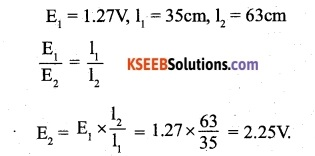Question 13.
The number density of free electrons in a copper conductor estimated in Example 3.1 is 8.5 x 1028 m-3. How long does an electron take to drift from one end of a wire 3.0 m long to its other end? The area of cross-section of the wire is 2.0 x’ 10-6 m2 and it is carrying a current of 3.0 A.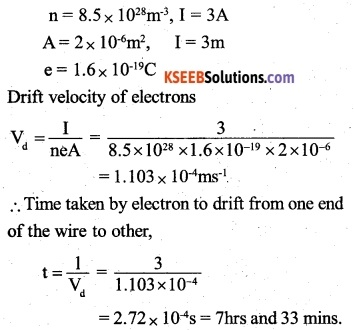### 2nd PUC Physics Current Electricity Additional Exercises

Question 14.
The earth’s surface has a negative surface charge density of 10-9 Cm-2. The potential difference of 400 kV between the top of the atmosphere and the surface results (due to the low conductivity of the lower atmosphere) in a current of only 1800 A over the entire globe. If there were no mechanism of sustaining atmospheric electric field, how much time (roughly) would be required to neutralise the earth’s surface? (This never happens in practice because there is a mechanism to replenish electric charges, namely the continual thunderstorms and lightning in different parts of the globe).
(Radius of earth = 6.37 x 10-6 m.)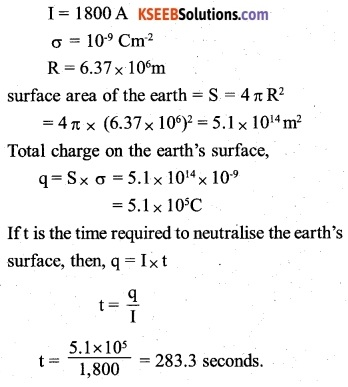Question 15.
(a) Six lead-acid type of secondary cells each of emf 2.0 V and internal resistance 0.015Ω are joined in series to provide a supply to a resistance of 8.5 Ω. What are the current drawn from the supply and its terminal voltage?

(b) A secondary cell after long use has an emf of 1.9 V and a large internal resistance of 380 Ω What maximum current can be drawn from the cell? Could the cell drive the starting motor of a car?
(a) When the cells are connected in series, the total emf is equal to sum of their emfs. Therefore, total emf of the cells,
E = 2 x 6=12v
Also, total internal resistance,
r = 0.015 x 6=0.09Ω
External resistance, R = 8.5 Ω
Therefore, current drawn from the supply,
$$I=\frac { E }{ R+r } =\frac { 12 }{ 85+0.09 } =1.397A$$
Terminal voltage, V=IR = 1.397 x8.5=11.875A

(b) Here, E = 1.9V; r = 380Ω
The maximum current that can be drawn corresponds to zero external resistance. Therefore
$${ I }_{ max }=\frac { 1.9 }{ 380 } =0.005A$$
But, a motor needs a current of 100 A or so for a few seconds. Hence, it cannot be used to drive the starting motor of a car.Question 16.
Two wires of equal length, one of aluminium and the other of copper have the same resistance. Which of the two wires is lighter? Hence explain why aluminium wires are preferred for overhead power cables.
AI= 2.63 x 10-8 Ωm, ρCu = 1.72 x 10-8 Ωm, Relative density of Al = 2.7, of Cu = 8.9.)
Let A1 and A2 be the area of cross-sections of the wires made from aluminium and copper respectively. Let R1 and R2, be the resistances of the same length 1 of the wires made of aluminium and copper respectively. For aluminium wire:

Density of the aluminium, d1 = 2.7 x 103 kgm-3 and resistivity of aluminium, ρal=2.63 x 108 Ωm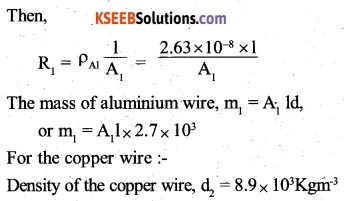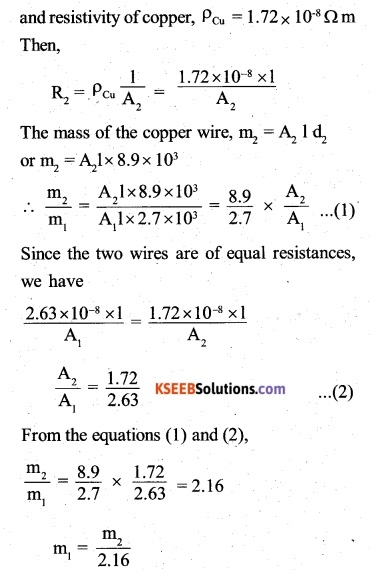It follows that the aluminium wire is lighter since for the same values of resistance and length, the aluminium wire has lesser mass, the aluminium wires are preferred for overhead power cables.

Question 17.
What conclusion can you draw from the following observations on a resistor made of alloy manganin?

 Current A Voltage V Current A Voltage V 0.2 3.94 3.0 59.2 0.4 7.87 4.0 78.8 0.6 11.8 5.0 98.6 0.8 15.7 6.0 118.5 1.0 19.7 7.0 138.2 2.0 39.4 8.0 158.0

It can be seen that for all the observations, the ratio of V to I is constant to fairly good
accuracy i.e. ohm’s law is obeyed accurately. Hence, the resistivity of alloy manganin is nearly independent of temperature.

Question 18.
Answer the following questions:
(a) A steady current flows in a metallic conductor of non-uniform cross-section. Which of these quantities is constant along the conductor: current, current density, electric field, drift speed?
(b) Is Ohm’s law universally applicable for all conducting elements? If not, give examples of elements which do not obey Ohm’s law.
(c) A low voltage supply from which one needs high currents must have very low internal resistance. Why?
(d) A high tension (HT) supply of, say, 6 kV must have a very large internal resistance. Why?
(a) Except current the values of all the other quantities depends upon the area of cross section of the conductor. Hence, only current remains constant, when it flows through a conductor of non-uniform area of cross­section.

(b) No, ohm’s law is not obeyed by all the elements. For example, vaccum diode tube and semiconductor diode.

(c) The maximum current that can be drawn from a voltage supply is given by,
$${ I }_{ max }=\frac { E }{ r }$$ Obviously, $${ I }_{ max }$$ will be large, if r is small.

(d) If the circuit containing the H.T supply gets short circuited accidently, the current in the circuit will not exceed the safe limit, in case the internal resistance of H.T supply is very large.Question 19.
Choose the correct alternative:
(a) Alloys of metals usually have (greater constituent metals.
(b) Alloys usually have much (lower/higher) temperature coefficients of resistance than pure metals.
(c) The resistivity of the alloy manganin is (nearly independent of/ increases rapidly with increase of) temperature.
(d) The resistivity of a typical insulator (e.g., amber) is greater than that of a metal by a factor of the order of (1022/103).
(a) greater
(b) lower
(c) nearly independent of
(d) 1022

Question 20.
(a) Given n resistors each of resistance R, how will you combine them to get the
(i) maximum
(ii) minimum effective resistance? What is the ratio of the maximum to minimum resistance?
(b) Given the resistances of 1Ω, 2Ω, 3Ω, how will combine them to get an equivalent resistance of
(j) (11/3)Ω
(ii) (11/5)Ω
(iii) (iv) (6/11)Ω
(c) Determine the equivalent resistance of networks shown in Fig. 3.31.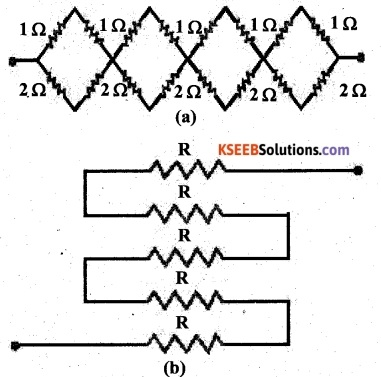(a) (i) The resistance will be maximum when resistors are connected in series. Therefore, maximum resistance.
$${ R }_{ max }$$ =R+R+R+……… n times = nR

(ii) The resistance will be minimum when resistors are connected in parallel. Therefore, minimum resistance,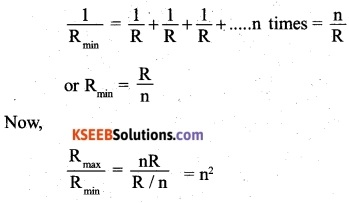b. (i) In order to obtain an equivalent resistance of 11/3 Ω the parallel combination of 1Ω and 2 Ω resistances should be connected in series to 3 Cl

(ii) In order to obtain an equivalent resistance of 11/5 Ω, the parallel combination of 2Ω and 3 Ω resistances should be connected in series to, 1 Ω resistance.

(iii) In order to obtain an equivalent resistance of 6Ω, this resistances should be connected in series.

(iv) In order to obtain an equivalent resistance of these resistance should be connected in parallel.

(c) (i)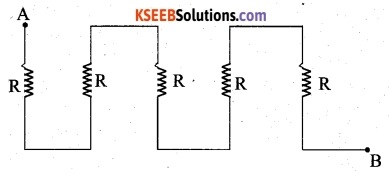If battery is connected across the points A and B, then the resistors are in series and hence the equivalent resistance will be the sum of all resistances,
i.e. $${ R }_{ eq }$$ = R + R + R + R + R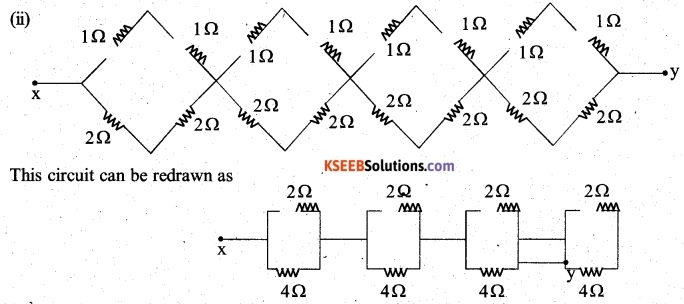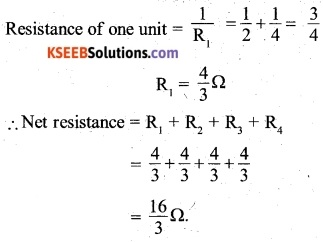Question 21.
Determine the current drawn from a 12V supply with internal resistance 0.5 Ω by the infinite network shown in Fig. 3.32. Each resistor has 1Ω resistance.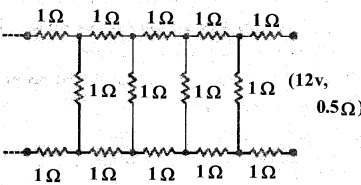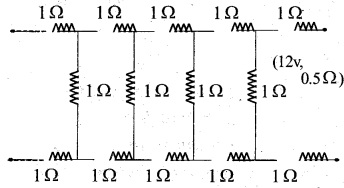Let the resistance equivalent to the entire network be X, Since the. network is infinite, adding one more set of three resistances each of value. R = 1 Ω across the terminals will not affect the total resistance i.e. it should still remain equal to X. Therefore, the network on adding one set of three resistances would appear as shown.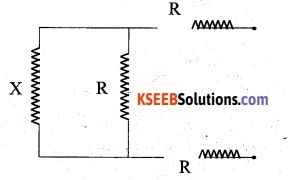If R’ is equivalent resistance of this new network, then = R + (resistance equivalent to parallel combination of X and R) + R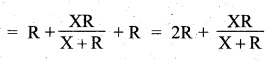As said above, the addition of one set of such three resistances should not alter the total resistance of the infinite network. Therefore,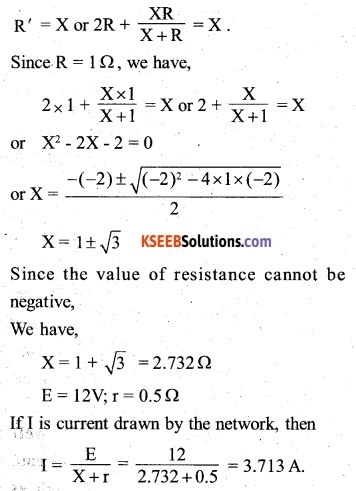Question 22.
Figure 3.33 shows a potentiometer with a cell of 2.0 V and internal resistance 0.40 £2 maintaining a potential drop across the resistor wire AB. A standard ceil which maintains a constant emf of 1.02 V (for very moderate currents upto a few mA) gives a balance point at 67.3 cm length of the wire. To ensure very low currents drawn from the standard cell, a very high resistance of 600 kΩ is put in series with it, which is shorted close to the balance point. The standard cell is then replaced by a cell of unknown emf g and the balance point found similarly, turns out to be at 82.3 cm length of the wire.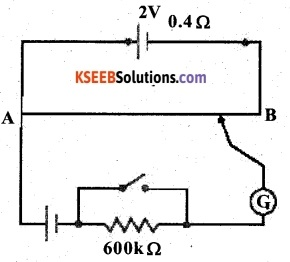(a) What is the value ε?
(b) What purpose does the high resistance of 600 kΩ have?
(c) Is the balance point affected by this high resistance?
(d) Is the balance point affected by the internal resistance of the driver cell?
(e) Would the method work in the above situation if the driver cell of the potentiometer had an emf of 1.0V instead of 2.0V?
(f) Would the circuit work well for determining an extremely small emf, say of the order of a few mV (such as the typical emf of a thermo-couple)? If not, how will you modify the circuit?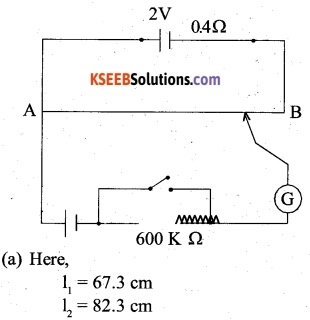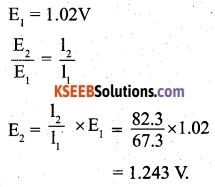(b) The purpose of using high resistance is to allow only a very small current to flow through the galvanometer when the balance point has not been obtained.
(c) No, the balance point is not affected by the internal resistance of the driver cell.
(d) No, the arrangement will not work. If the emf of the driver cell is less than that of the cell whose emf is to be found, the balance point will not be obtained.
(e) The circuit is not suitable for measuring extremely small emf. It is because in such a case, the balance point will be just close to end A.

Question 23.
Figure 3.34 shows a potentiometer circuit for comparison of two resistances. The balance point with a standard resistor R = 10.0 Ω is found to be 58.3 cm, while that with the unknown resistance X is 68.5 cm. Determine the value of X. What might you do if you failed to find a balance point with the given cell of emf ε?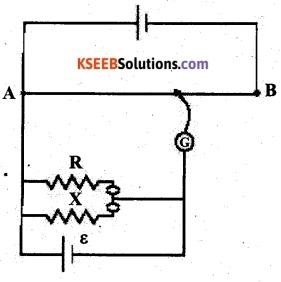(a) Let E1 and E2 be the potential drops across R and X respectively.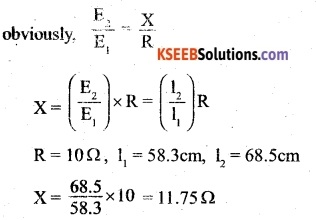(b) We fail to get the balance point with the given cell of emf E, if the potential difference across the wire AB. In order to obtain the balance point with the given cell E, either the emf of the auxiliary7 battery (between A and B) should be increased or a suitable resistance should be put in series with R and X (so as to decrease the potential drop across the wire AB).Question 24.
Figure 3.35 shows a 2.0 V potentiometer Used for the determination of the internal resistance of a 1.5 V cell. The balance point of the cell in open circuit is 76.3 cm. When a resistor of 9.5 Ω is used in the external circuit of the cell, the balance point shifts to 64.8 cm length of the potentiometer wire. Determine the internal resistance of the cell.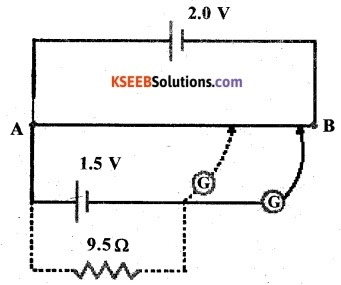It follows that the emf of the cell E is balanced against the potential difference across the length $${ I }_{ 1 }$$ and the terminal potential difference V against the potential difference across the length $${ I }_{ 2 }$$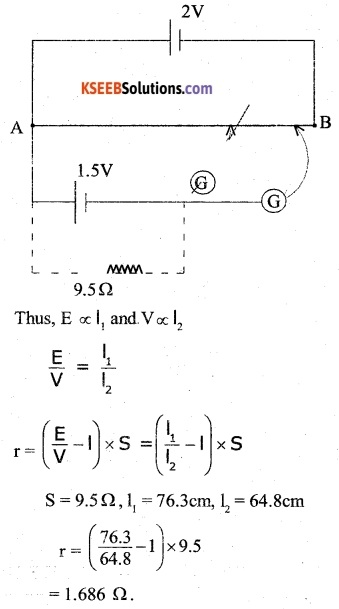### 2nd PUC Physics Current Electricity Entrance Examination Questions and Answers

Question 1.
The length of a given cylindrical wire is increased by 100%. Due to the consequent decrease in diameter the change in the resistance of the wire will be
(A) 200%
(B) 100%
(C) 50%
(D) 300%.
(D) 300%.

Question 2.
The total current supplied to the circuit by the battery is
(A) 1 A
(B) 2A
(C) 4 A
(D) 6 A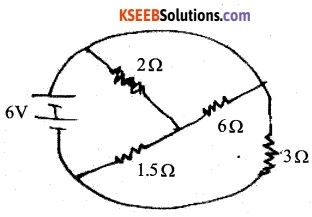(C) 4 A

Question 3.
What will be the value of current through 2 resistance for the circuit shown in the figure.
(A) 5A
(B) 2A
(C) Zero
(D) 4 A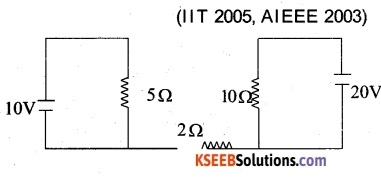(C) Zero

Question 4.
Kirchoff’sfirst law, Σ I = 0 at a junction, deals with the conservation of (CBSE 1997)
(A) Charge
(B) Energy
(C) Momentum
(D) Angular momentum
(A) Charge

Question 5.
For the network k shown in the figure, the value of the current I is (CBSE 2005)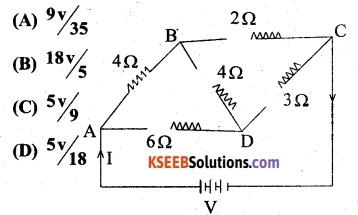(D)

Question 6.
What will be the equilibrium resistance between points A&D
(A) 10 Ω
(B) 20 Ω
(C) 30 Ω
(D) 40Ω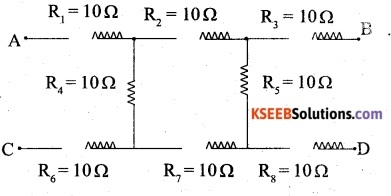(C) 30 Ω

Question 7.
What is the net resistance between A and B?(AIEEE 2008)
(A) R
(B) 5R
(C) 3R
(D) 6R